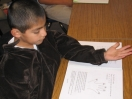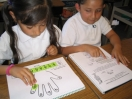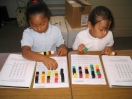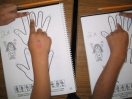How Han-5 workbooks teach students how to solve any kind of math problem with the Han-5 System of Mathematics in three simple steps.First, the workbook teaches the repetitive numbers for the one's place (Han-5 names these numbers base numbers), using one hand as a visual representation. (for the visual learners)Next, the workbook shows students when to add sequential tens (10, 20, 30, etc...) to these repetitive base numbers. (for the visual learners)Then listen to funny stories about frogs and fish, while placing sequential tens under these base numbers and adding them together on your Han-5 Boogie Boards. (for the auditory and kinesthetic learners)

After these two steps the visual, kinesthetic, and auditory learners are all empowered to speak by multiples within minutes.Next the workbook shows students how the Han-5 System vacuums a hundred chart, a number line and...Multiplication and division tables onto one hand. Learning Han-5 simplifies learning math facts, shows relationships between math fact families and demonstrates the conceptual understanding of multiplication and division.Then the workbook shows how simple it is to multiple, divide, divide uneven numbers, write equivalent fractions, solve parts to whole, ratios, algebra, prime factorization, finding the L.C.M. and G.C.F., solving for squares and square roots, and reducing fractions just by learning Han-5.)